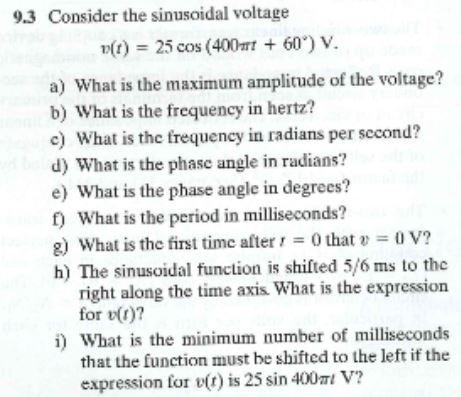# (Solved): I have solved A-G. Can you please solve h and i for me? Thanks! $$9.3$$ Consider the sinuso ...I have solved A-G. Can you please solve h and i for me? Thanks!

$$9.3$$ Consider the sinusoidal voltage $v(t)=25 \cos \left(400 \pi t+60^{\circ}\right) \mathrm{V} .$ a) What is the maximum amplitude of the voltage? b) What is the frequency in hertz? c) What is the frequency in radians per sccond? d) What is the phase angle in radians? e) What is the phase angle in degrees? f) What is the period in milliseconds? g) What is the first time after $$t=0$$ that $$v=0 \mathrm{~V}$$ ? h) The sinusoidal function is shifted $$5 / 6 \mathrm{~ms}$$ to the right along the time axis. What is the expression for $$v(t)$$ ? i) What is the minimum number of milliseconds that the function must be shifted to the left if the expression for $$v(t)$$ is $$25 \sin 400 \pi t \mathrm{~V}$$ ?

We have an Answer from Expert